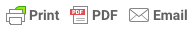# College CatalogGPA is computed by dividing the total units attempted (including units for courses repeated) into the total grade points. “P”, “NP”, “CR,”  “NC,”  “I,” and “W” are not included in the total course units attempted. For example, a student who earns a grade of “A” in six units, “B” in three units, “C” in three units, “D” in three units, and “F” in two and one-half units would have a GPA of 2.40.

The computation is shown here: